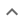•We think you wrote:

###This solution deals with quadratic equations.

Solution foundx=(4-sqrt(-8))/2=2-isqrt(2)=2.0000-1.4142ix=(4+sqrt(-8))/2=2+isqrt(2)=2.0000+1.4142i

## Step by Step Solution### Reformatting the input :

(1): "x2"   was replaced by   "x^2".

### Rearrange:

Rearrange the equation by subtracting what is to the right of the equal sign from both sides of the equation :

x^2-4*x-(-6)=0

## Step  1  :

#### Trying to factor by splitting the middle term

Factoring  x2-4x+6

The first term is,  x2  its coefficient is  1 .
The middle term is,  -4x  its coefficient is  -4 .
The last term, "the constant", is  +6

Step-1 : Multiply the coefficient of the first term by the constant   1 • 6 = 6

Step-2 : Find two factors of  6  whose sum equals the coefficient of the middle term, which is   -4 .

 -6 + -1 = -7 -3 + -2 = -5 -2 + -3 = -5 -1 + -6 = -7 1 + 6 = 7 2 + 3 = 5 3 + 2 = 5 6 + 1 = 7

Observation : No two such factors can be found !!
Conclusion : Trinomial can not be factored

#### Equation at the end of step  1  :

  x2 - 4x + 6  = 0


## Step  2  :

#### Parabola, Finding the Vertex :

Find the Vertex of   y = x2-4x+6

Parabolas have a highest or a lowest point called the Vertex .   Our parabola opens up and accordingly has a lowest point (AKA absolute minimum) .   We know this even before plotting  "y"  because the coefficient of the first term, 1 , is positive (greater than zero).

Each parabola has a vertical line of symmetry that passes through its vertex. Because of this symmetry, the line of symmetry would, for example, pass through the midpoint of the two  x -intercepts (roots or solutions) of the parabola. That is, if the parabola has indeed two real solutions.

Parabolas can model many real life situations, such as the height above ground, of an object thrown upward, after some period of time. The vertex of the parabola can provide us with information, such as the maximum height that object, thrown upwards, can reach. For this reason we want to be able to find the coordinates of the vertex.

For any parabola,Ax2+Bx+C,the  x -coordinate of the vertex is given by  -B/(2A) . In our case the  x  coordinate is   2.0000

Plugging into the parabola formula   2.0000  for  x  we can calculate the  y -coordinate :

y = 1.0 * 2.00 * 2.00 - 4.0 * 2.00 + 6.0
or   y = 2.000

#### Parabola, Graphing Vertex and X-Intercepts :

Root plot for :  y = x2-4x+6
Axis of Symmetry (dashed)  {x}={ 2.00}
Vertex at  {x,y} = { 2.00, 2.00}
Function has no real roots

#### Solve Quadratic Equation by Completing The Square

Solving   x2-4x+6 = 0 by Completing The Square .

Subtract  6  from both side of the equation :
x2-4x = -6

Now the clever bit: Take the coefficient of  x , which is  4 , divide by two, giving  2 , and finally square it giving  4

Add  4  to both sides of the equation :
On the right hand side we have :
-6  +  4    or,  (-6/1)+(4/1)
The common denominator of the two fractions is  1   Adding  (-6/1)+(4/1)  gives  -2/1
So adding to both sides we finally get :
x2-4x+4 = -2

Adding  4  has completed the left hand side into a perfect square :
x2-4x+4  =
(x-2) • (x-2)  =
(x-2)2
Things which are equal to the same thing are also equal to one another. Since
x2-4x+4 = -2 and
x2-4x+4 = (x-2)2
then, according to the law of transitivity,
(x-2)2 = -2

We'll refer to this Equation as  Eq. #2.2.1

The Square Root Principle says that When two things are equal, their square roots are equal.

Note that the square root of
(x-2)2   is
(x-2)2/2 =
(x-2)1 =
x-2

Now, applying the Square Root Principle to  Eq. #2.2.1  we get:
x-2 = -2

Add  2  to both sides to obtain:
x = 2 + √ -2
In Math,  i  is called the imaginary unit. It satisfies   i2  =-1. Both   i   and   -i   are the square roots of   -1

Since a square root has two values, one positive and the other negative
x2 - 4x + 6 = 0
has two solutions:
x = 2 + √ 2  i
or
x = 2 - √ 2  i

Solving    x2-4x+6 = 0 by the Quadratic Formula .

According to the Quadratic Formula,  x  , the solution for   Ax2+Bx+C  = 0  , where  A, B  and  C  are numbers, often called coefficients, is given by :

- B  ±  √ B2-4AC
x =   ————————
2A

In our case,  A   =     1
B   =    -4
C   =   6

Accordingly,  B2  -  4AC   =
16 - 24 =
-8

4 ± √ -8
x  =    —————
2

In the set of real numbers, negative numbers do not have square roots. A new set of numbers, called complex, was invented so that negative numbers would have a square root. These numbers are written  (a+b*i)

Both   i   and   -i   are the square roots of minus 1

Accordingly, -8  =
√ 8 • (-1)  =
√ 8  • √ -1   =
±  √ 8  • i

Can  √ 8 be simplified ?

Yes!   The prime factorization of  8   is
2•2•2
To be able to remove something from under the radical, there have to be  2  instances of it (because we are taking a square i.e. second root).

8   =  √ 2•2•2   =
±  2 • √ 2

√ 2   , rounded to 4 decimal digits, is   1.4142
So now we are looking at:
x  =  ( 4 ± 2 •  1.414 i ) / 2

Two imaginary solutions :

 x =(4+√-8)/2=2+i√ 2 = 2.0000+1.4142i
or:  x =(4-√-8)/2=2-i√ 2 = 2.0000-1.4142i


## Two solutions were found :

1.  x =(4-√-8)/2=2-i 2 = 2.0000-1.4142i
2.  x =(4+√-8)/2=2+i 2 = 2.0000+1.4142i